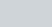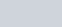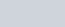Prove that the points (4, 5) (7, 6), (6, 3) (3, 2)
Question:

Prove that the points (4, 5) (7, 6), (6, 3) (3, 2) are the vertices of a parallelogram. Is it a rectangle.

Solution:

Let A (4, 5); B (7, 6); C (6, 3) and D (3, 2) be the vertices of a quadrilateral. We have to prove that the quadrilateral ABCD is a parallelogram.

We should proceed with the fact that if the diagonals of a quadrilateral bisect each other than the quadrilateral is a parallelogram.

Now to find the mid-pointof two pointsandwe use section formula as,

$\mathrm{P}(x, y)=\left(\frac{x_{1}+x_{2}}{2}, \frac{y_{1}+y_{2}}{2}\right)$

So the mid-point of the diagonal AC is,

$\mathrm{Q}(x, y)=\left(\frac{4+6}{2}, \frac{5+3}{2}\right)$

$=(5,4)$

Similarly mid-point of diagonal BD is,

$R(x, y)=\left(\frac{7+3}{2}, \frac{6+2}{2}\right)$

$=(5,4)$

Therefore the mid-points of the diagonals are coinciding and thus diagonal bisects each other.

Hence ABCD is a parallelogram.

Now to check if ABCD is a rectangle, we should check the diagonal length.

$\mathrm{AC}=\sqrt{(6-4)^{2}+(3-5)^{2}}$

$=\sqrt{4+4}$

$=2 \sqrt{2}$

Similarly,

$\mathrm{BD}=\sqrt{(7-3)^{2}+(6-2)^{2}}$

$=\sqrt{16+16}$

$=4 \sqrt{2}$

Diagonals are of different lengths.

Hence ABCD is not a rectangle.